## 2.5 Visualization

A graph is a set of points. A mathematical graph cannot be seen. It is an abstraction. A graphic, however, is a physical representation of a graph. This representation is accomplished by realizing graphs with aesthetic attributes such as size or color.

Visualization is the process of representing information or ideas through diagrams, graphics, and other methods of visual presentation. In general, visualization tools should be clear to the reader and unnecessary details should be avoided. A good viewer conveys the desired information clearly, accurately, and efficiently.

According to , besides “forty years of thought and achievement in the Indian question” , “safeguarding the health of the British soldier”, “reorganizing civil and military hospital administration at home and abroad” and “her pioneer services to the profession of nursing”, the activities of Florence Nightingale in statistics “may be classed under several broad categories”. “The Lady with the Lamp”, a “passionate statistician” according , popularized the polar-area diagram, what she called “coxcombs” . Moreover, she did an outstanding job on the visualization part in documenting information relating to the war fronts .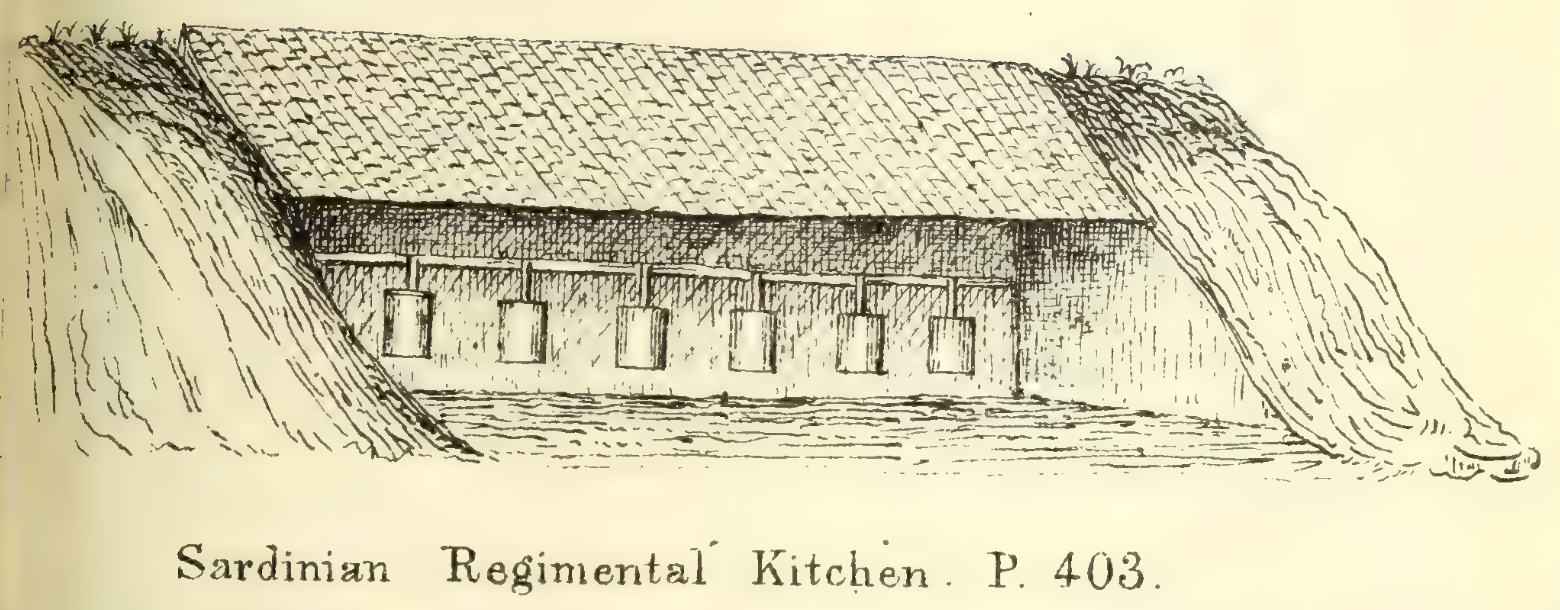Edward Tufte, “the Leonardo da Vinci of data” according to The New York Times, or “the Galileo of graphics”, according to Bloomberg, has a vast body of work on the subject, highlighting , , , and . More recently, visual artists such as Karim Douieb13 can be found, who has a considerable published portfolio.

Some principles:

• less is more
• keep the notation consistent with the text
• the human eye does not differentiate many shades of color
• work with gradient scales, different textures and line weights

### 2.5.1 Examples

Example 2.41 Visualization considering the aforementioned principles.

It is these 109 billion people we have to thank for the civilization that we live in. The languages we speak, the food we cook, the music we enjoy, the tools we use – what we know we learned from them.
Max Roser (2022-03-15)

Example 2.42 Land doesn’t vote. People do.Example 2.43 Would bicycles be the transport of the future invented in the past?

tweetrmd::include_tweet('https://twitter.com/Twouttter/status/1635941366747258883?s=20')

Example 2.44 According to , if there are three candidates on the verge of a technical tie according to the method adopted by research institutes – i.e., $$A$$ ties with $$B$$ by one point, $$B$$ ties with $$C$$ by a point but $$A$$ and $$C$$ do not tie (??) –, the blue ellipse in the simplex below indicates the likely electoral scenarios with a sample size of 500. In this case, there must be a second round between $$A$$ and $B$.

desempateTecnico::simplex3d(.3919345050, .3324785813, .2755869137, 500)
rgl::rglwidget()

### 2.5.2 Basic charts

#### 2.5.2.1 Pie

The idea is to draw sectors/slices proportional to the frequencies of the categories. Following the graphic presentation etiquette, it is recommended to use this type of graphic for a maximum of ten categories. Also, by default displayed counterclockwise starting at 0°.

atend <- c(90,62,31,44,13)                      # Number of attendances
colors <- gray(0:4/4)                           # Five shades of gray
atend_rel <- round(atend/sum(atend) * 100, 1)   # Calculating the percentages
atend_rel <- paste(atend_rel, '%', sep='')      # Adding '%'

# Frequency
pie(atend, main = 'Attendances', col = colors, labels = atend,
cex = 1.2, cex.main = 1.7)
legend(1.1, 0.9, c('Mon','Tue','Wed','Thu','Fri'), cex = 1.3, fill = colors,
box.col = 'white')# Relative frequency
pie(atend, main = 'Attendances', col = colors, labels = atend_rel,
cex = 1.2, cex.main = 1.7)
legend(1.3, 0.9, c('Mon','Tue','Wed','Thu','Fri'), cex = 1.3, fill = colors,
box.col='white')#### 2.5.2.2 Bars and Columns

The bar chart is typically used to display data sorted into unordered categories. Rectangular bars of the same width are placed over the categories with height proportional to the frequencies or other measure associated with the categories. They can be arranged horizontally or vertically; when grouped in the latter way, it is called a column chart. It is a very diversified graph, as it allows you to represent information in different ways.

# Data
expenses <- c(760, 640, 75, 850, 100)
names(expenses) <- c('education', 'food', 'electricity', 'housing', 'internet')

# Bars
barplot(expenses, xlab = 'Expenses (R$)', main = 'Personal expenses', col = gray(0:4/4), las = 0, cex.main = 1.6, horiz = TRUE)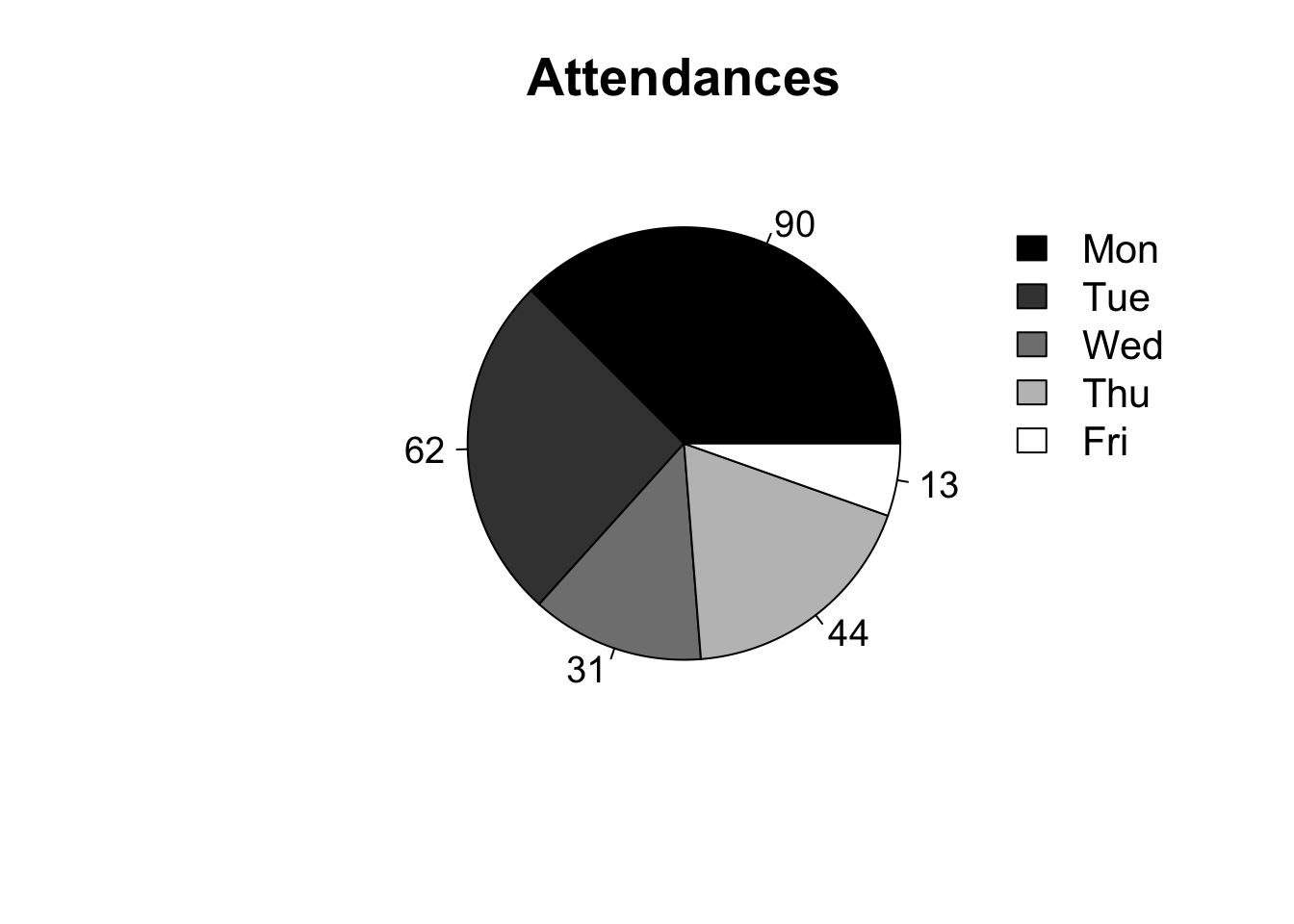# Columns barplot(expenses, ylab = 'Expenses (R$)', main = 'Personal expenses',
col = gray(0:4/4), las = 0, cex.main = 1.6)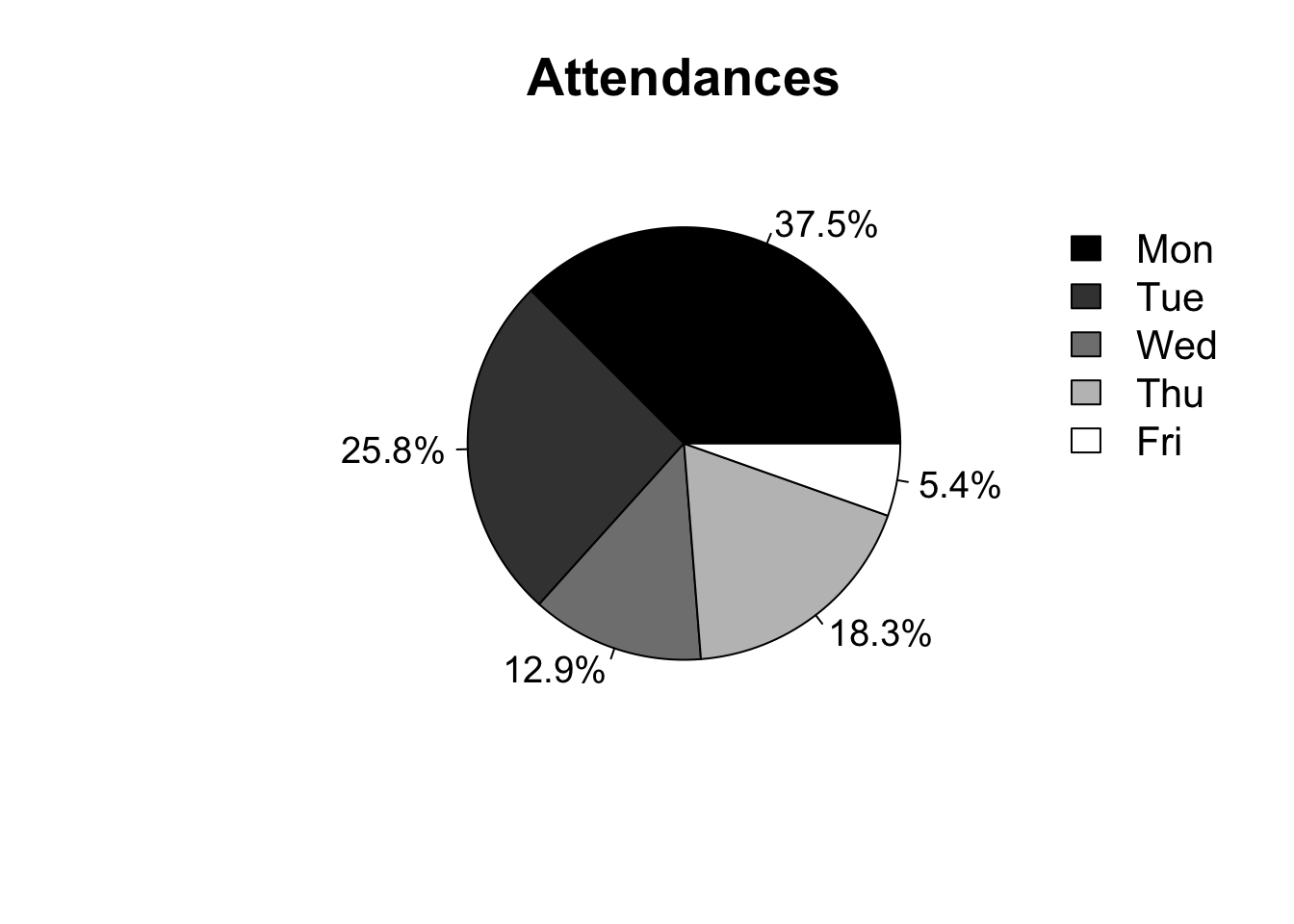# Stacked columns
library(vcd)
tr <- table(Arthritis$Improved, Arthritis$Treatment)
rownames(tr) <- c('No improvement', 'Some improvement', 'Full improvement')
colnames(tr) <- c('Placebo', 'Treatment')

barplot(tr,
main = 'Placebo vs Treatment',
ylab = 'Frequency',
col = c('black', 'grey', 'white'),
cex.main = 1.6)
legend(1.5, 40, rownames(tr), cex = 1, fill = colors[c(1,3,5)],
box.col = 'white')# Columns side by side
barplot(tr,
main = 'Placebo vs Treatment',
ylab = 'Frequency',
col = c('black', 'grey', 'white'),
cex.main = 1.6, beside = TRUE)
legend(4, 28, rownames(tr), cex = 1, fill = colors[c(1,3,5)],
box.col = 'white')#### 2.5.2.3 Histogram

The histogram is the classical nonparametric density estimator, probably dating from the mortality studies of John Graunt in 1662.

The histogram is a bar graph without spacing used to represent frequency distributions of continuous variables. The term was introduced by Karl Pearson “in his lectures on statistics as a term for a common form of graphical representation, i.e., by columns marking as areas the frequency corresponding to the range of their base”.

The variable divided into classes on the horizontal axis ($$x$$) and the frequency of each class on the vertical axis ($$y$$) are presented. Computational packages in general define the number of classes by the Sturges rule according to Eq. (2.3). It is a basic exploratory data analysis tool to assess data dispersion and shape, detect outliers, and suggest models and transformations for more advanced analysis.

# Data

# Standard histogram
hist(h$height, prob = FALSE, right = FALSE, breaks = 'sturges', main = '', xlab = 'Height (m)', col = 'grey')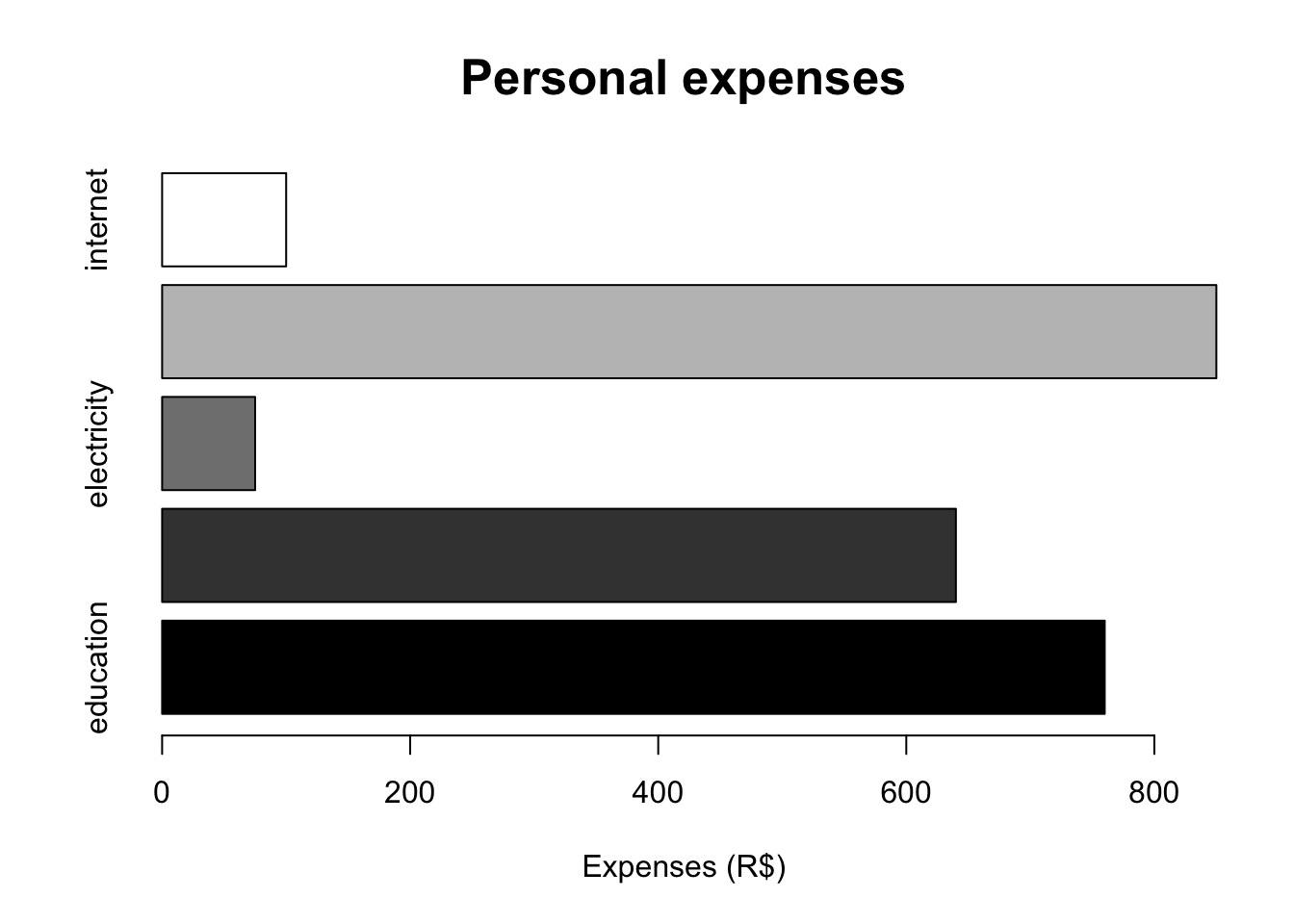# Freedman-Diaconis class interval hist(h$height, prob = TRUE, right = FALSE, breaks = 'fd', main = '',
xlab = 'Height (m)', col = 'grey')
curve(dnorm(x, mean = mean(h$height), sd = sd(h$height)), col = 'blue', lwd = 2,
add = TRUE)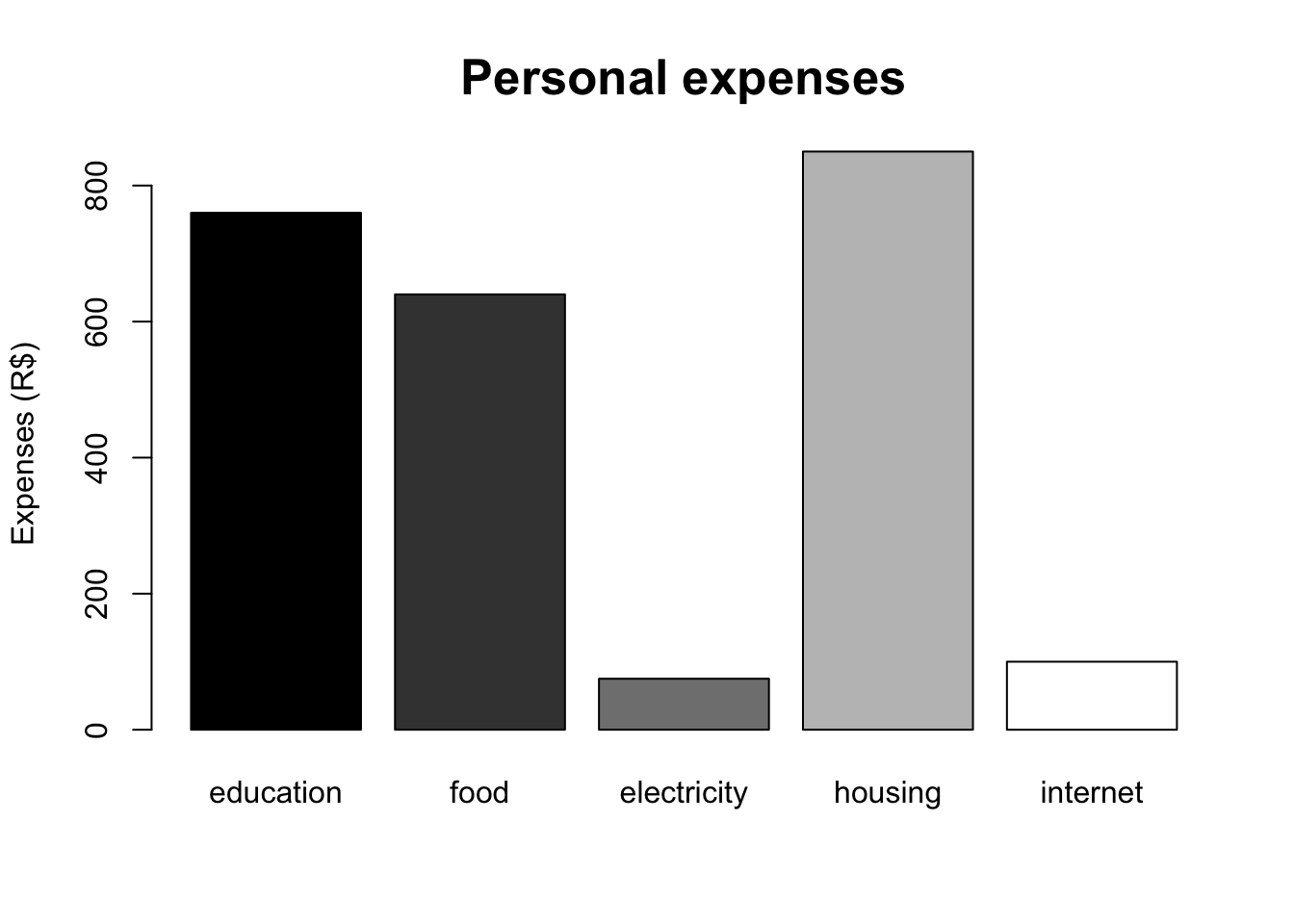# Using the ggplot2 library
ggplot2::qplot(h$height, geom = 'histogram', xlab = 'Height (m)')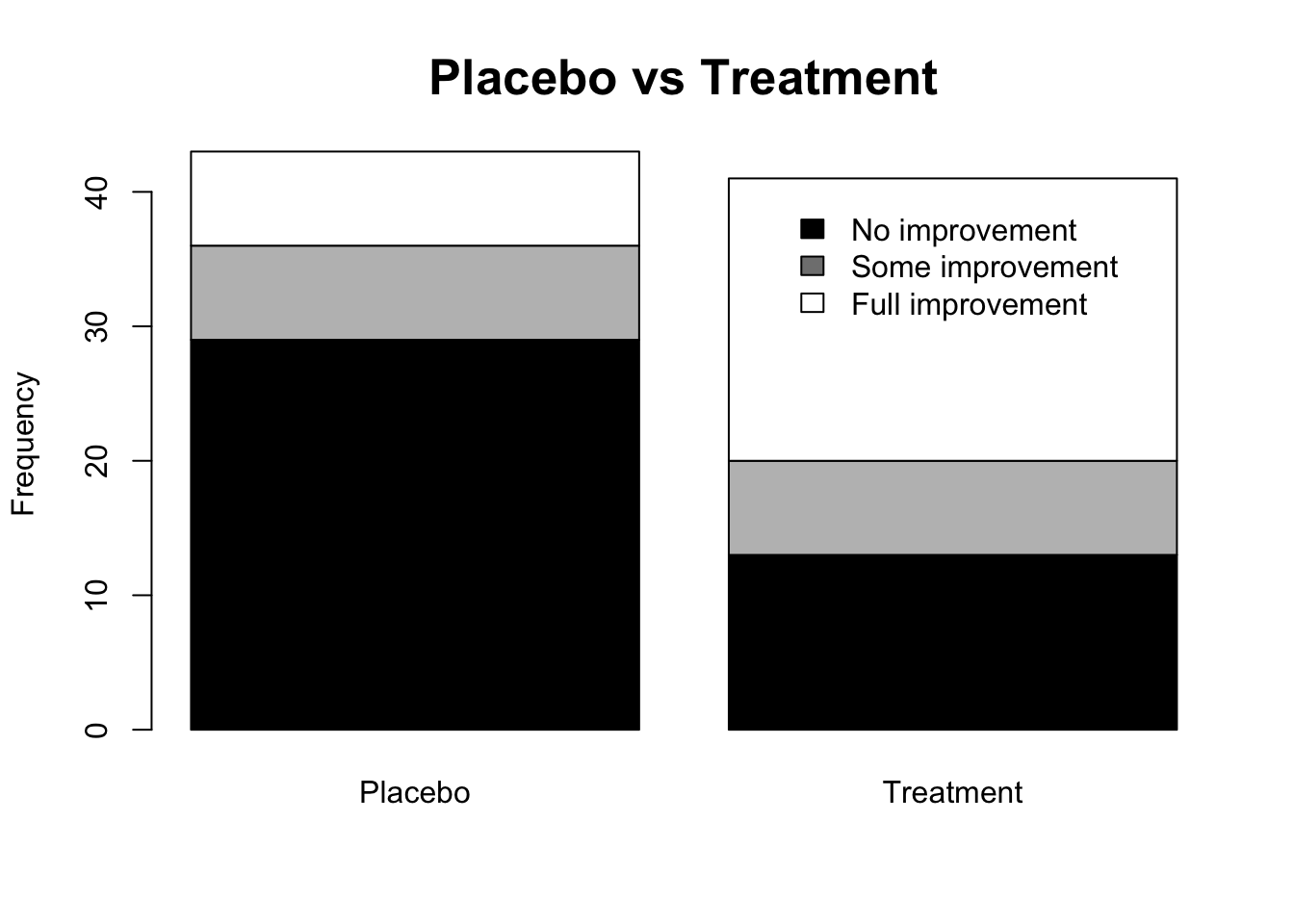#### 2.5.2.4 Boxplot Introduced by , the boxplot is a graph in rectangular format bounded by the first and third quartiles, where the center line is the median. The distance between quartiles is the interquartile range as per Section 2.4.4 and includes $$50\%$$ of core data. Points exceeding $$1.5$$ times the interquartile range above (below) $$Q_{3}$$ ($$Q_{1}$$) are called outliers. Variations are discussed by , and . # dados h <- read.csv('https://filipezabala.com/data/hospital.csv', header = TRUE) # Boxplot boxplot(h$children, main = 'Children', ylab = 'Children',
las = 1, cex.main = 1.6)
legend(1.32, 0.1, 'Minimum', box.col = 'white')
arrows(x0 = 1.35, y0 = 0, x1 = 1.25, y1 = 0, length = 0.15)
legend(1.32, 1.1, 'Q1', box.col = 'white')
arrows(x0 = 1.35, y0 = 1, x1 = 1.25, y1 = 1, length = 0.15)
legend(1.32, 2.1, 'Median', box.col = 'white')
arrows(x0 = 1.35, y0 = 2, x1 = 1.25, y1 = 2, length = 0.15)
legend(1.32, 3.1, 'Q3', box.col='white')
arrows(x0 = 1.35, y0 = 3, x1 = 1.25, y1 = 3, length = 0.15)
legend(1.32, 6.1, 'Maximum', box.col = 'white')
arrows(x0 = 1.35, y0 = 6, x1 = 1.25, y1 = 6, length = 0.15)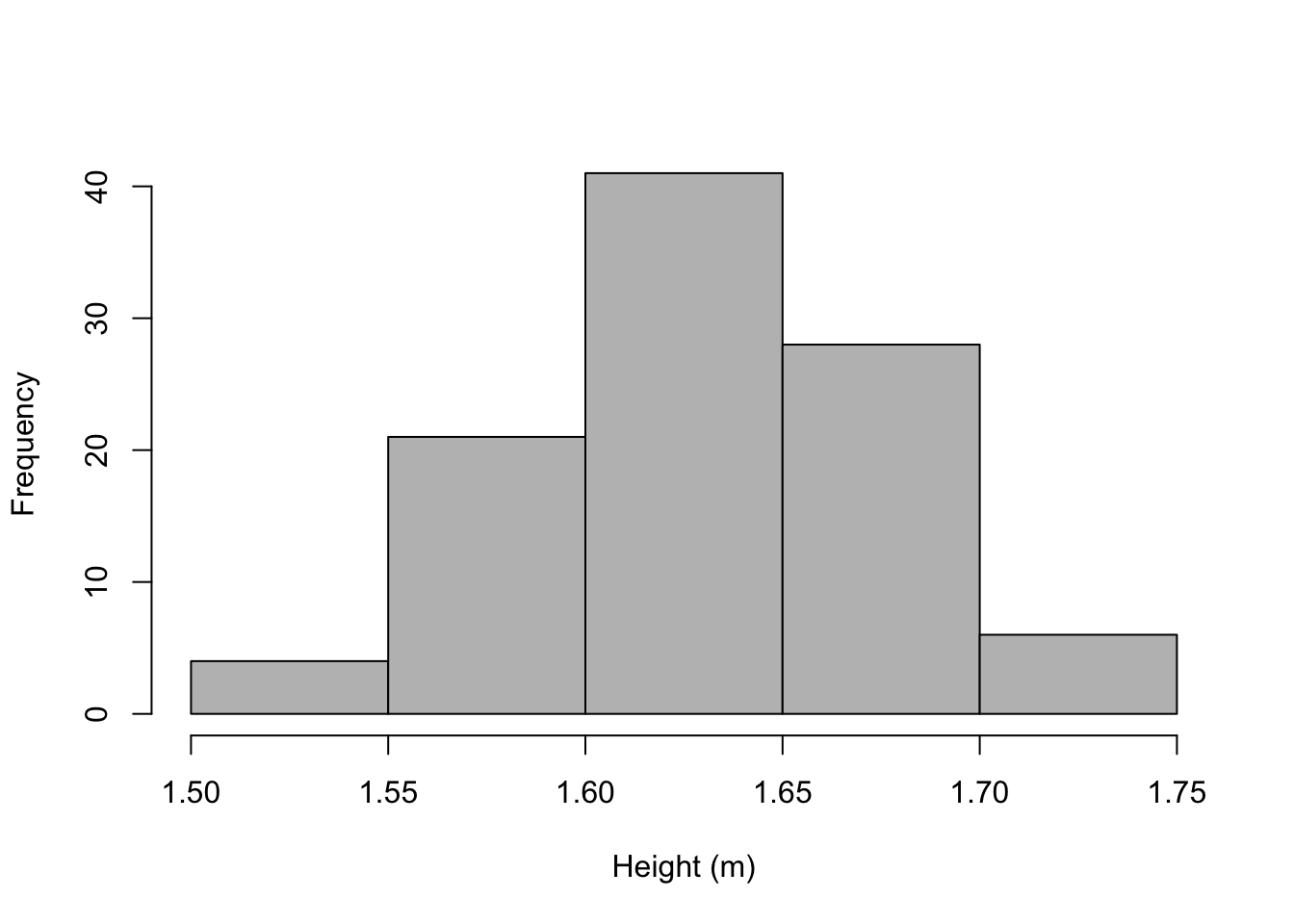# Proportional to group size
set.seed(1); y <- c(rpois(50, lambda=1.5), rnorm(300,4), (1:150)/17)
x <- factor(c(rep('A',50), rep('B',300), rep('C',150) ))
bp <- boxplot(y ~ x, varwidth = TRUE, las = TRUE, main = 'Variable W',
cex.main = 1.6)
mtext(paste('(n=', bp$n, ')', sep = ''), at = seq_along(bp$n), line = 2,
side = 1)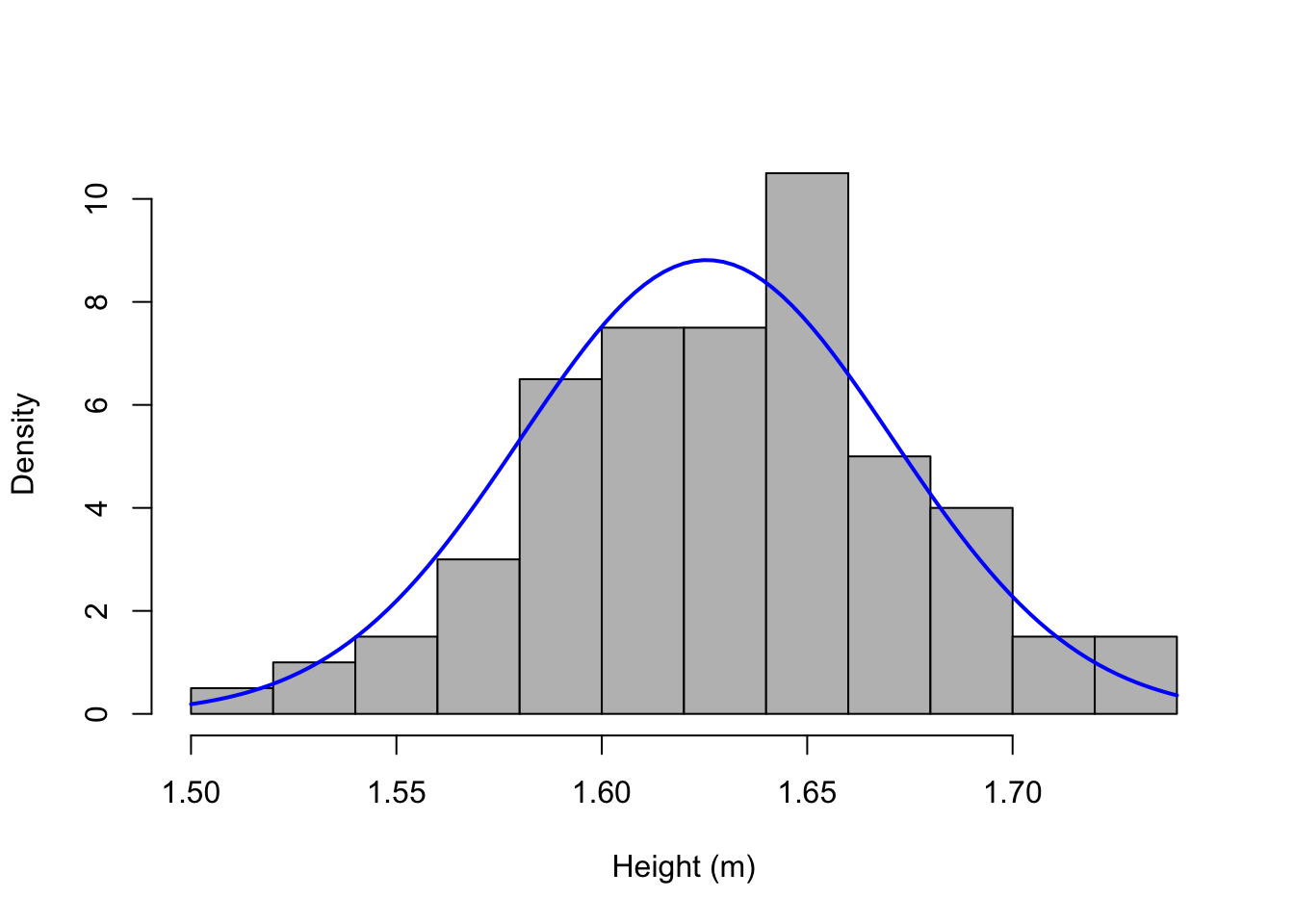# Vertical
boxplot(count ~ spray, data = InsectSprays, col = 'lightgray',
main = 'Score on a test', ylab = 'Score', xlab = 'Group',
las = 1, cex.main = 1.6)
legend(2.85, 18.5, 'Outliers', box.col = 'white')
arrows(x0 = 3.4,y0 = 15, x1 = 3.05, y1 = 7.8, length = 0.15)
arrows(x0 = 3.4,y0 = 15, x1 = 3.9, y1 = 12.3, length = 0.15)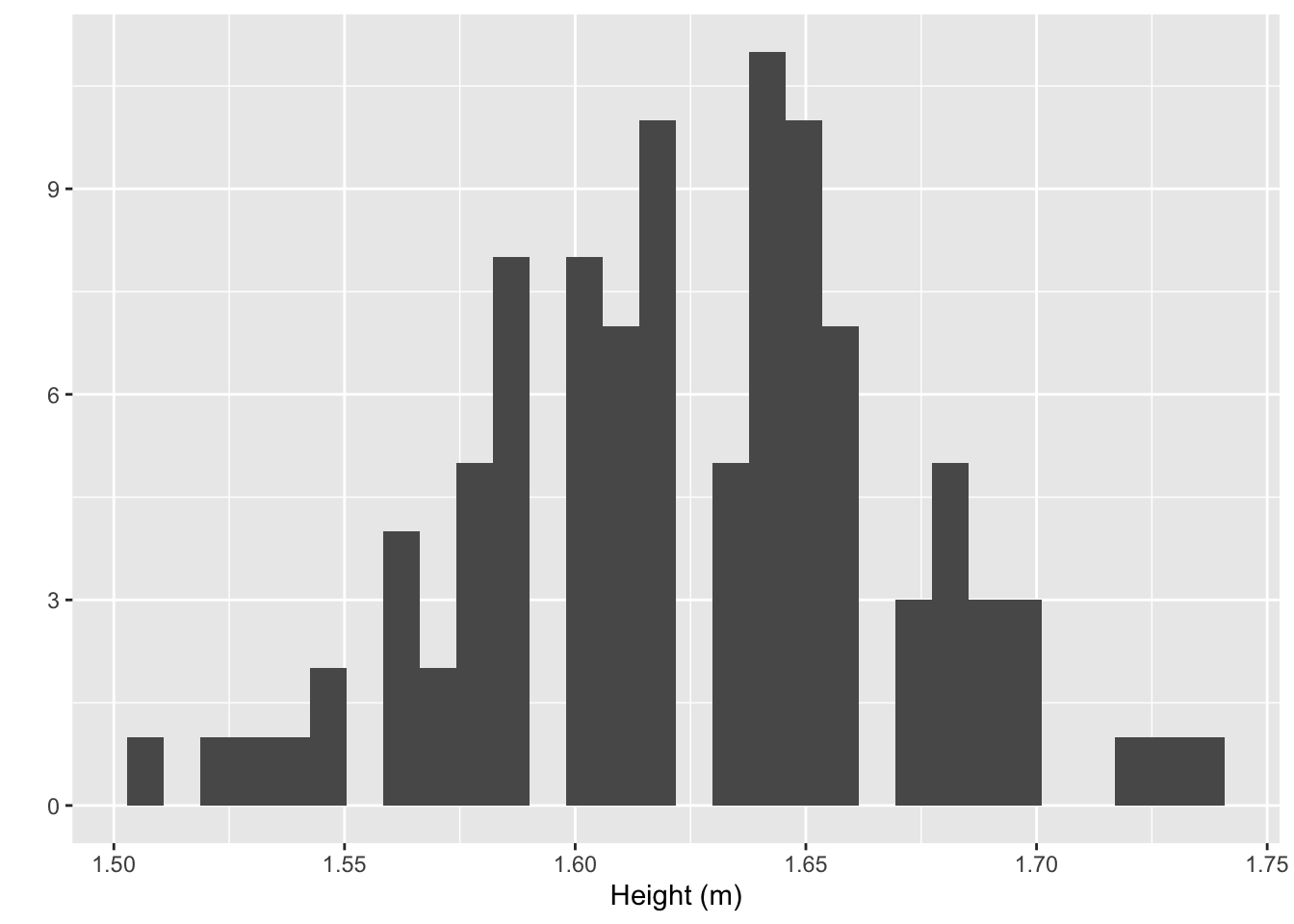# Horizontal
boxplot(count ~ spray, data = InsectSprays, col = 'lightgray',
main = 'Score on a test', ylab = 'Score', xlab = 'Group',
las = 1, cex.main = 1.6, horizontal = TRUE)#### 2.5.2.5 Scatter plot

The scatter plot shows the relationship between two numeric variables. It is a useful tool for adjusting the models presented in Chapter 7.

performance <- 0.42515199183708*mtcars$mpg weight <- 0.453592*mtcars$wt*1000
displacement <- 16.387064*mtcars$disp rear_axle_ratio <- mtcars$drat

# Scatter plot
plot(weight, performance,
main = 'Performance (km/L) vs Weight (kg)',
xlab = 'Car weight (kg)',
ylab = 'Performance (km/L)',
pch = 19, las = 1, cex.main = 1.6)# Scatter matrix
pairs(~ performance + weight + displacement + rear_axle_ratio,
main = 'Scatter matrix', cex.main = 1.6)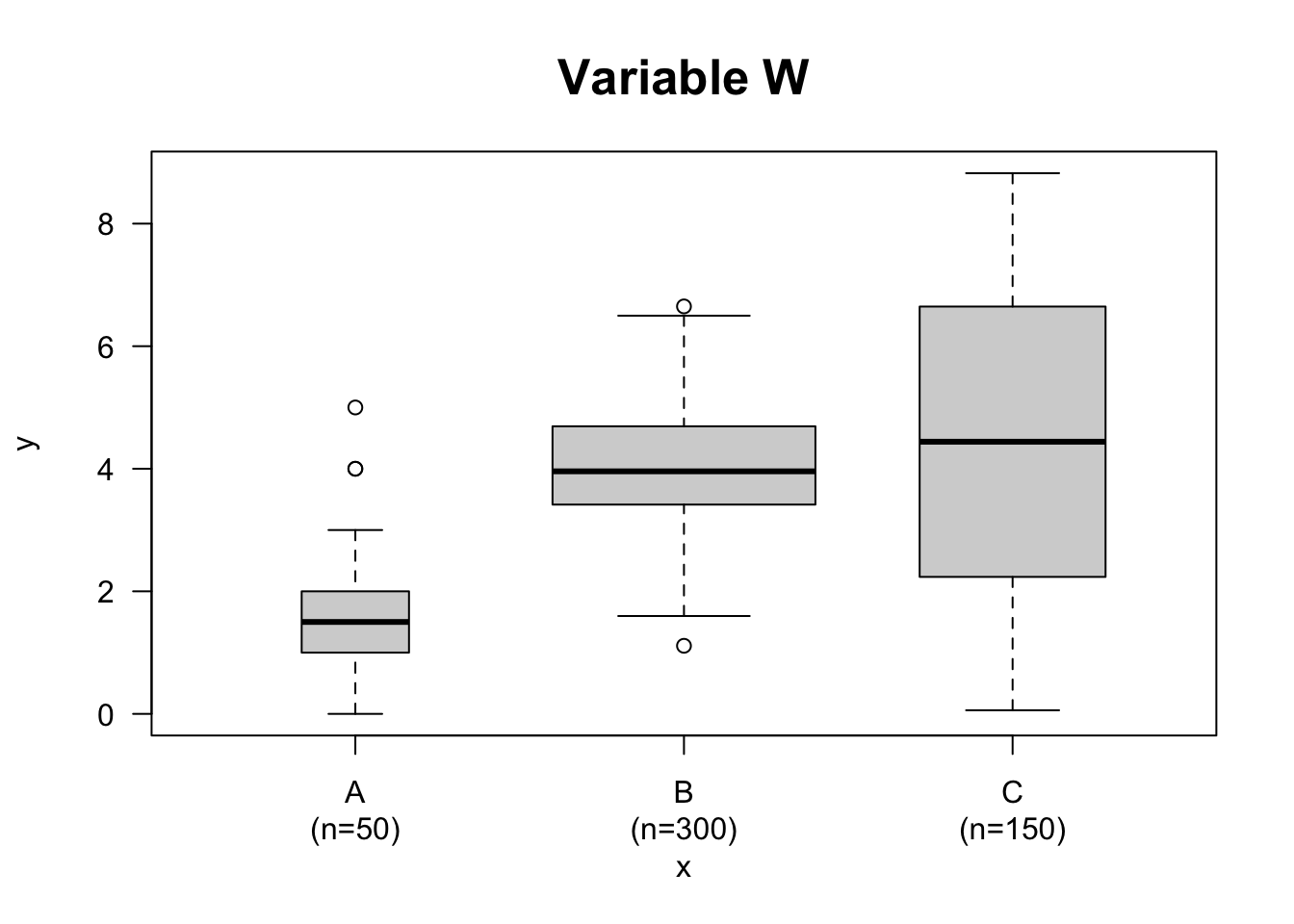#### 2.5.2.6 Correlogram

# install.packages(c('ellipse','RColorBrewer'), dep=T)
library(ellipse)
library(RColorBrewer)

# Using the 'mtcars' database
data <- cor(mtcars)

# 100 color panel with Rcolor Brewer
my_colors <- brewer.pal(5, "Spectral")
my_colors <- colorRampPalette(my_colors)(100)

# Sorting the correlation matrix
ord <- order(data[1, ])
data_ord <- data[ord, ord]
plotcorr(data_ord , col=my_colors[data_ord*50+50] , mar=c(1,1,1,1))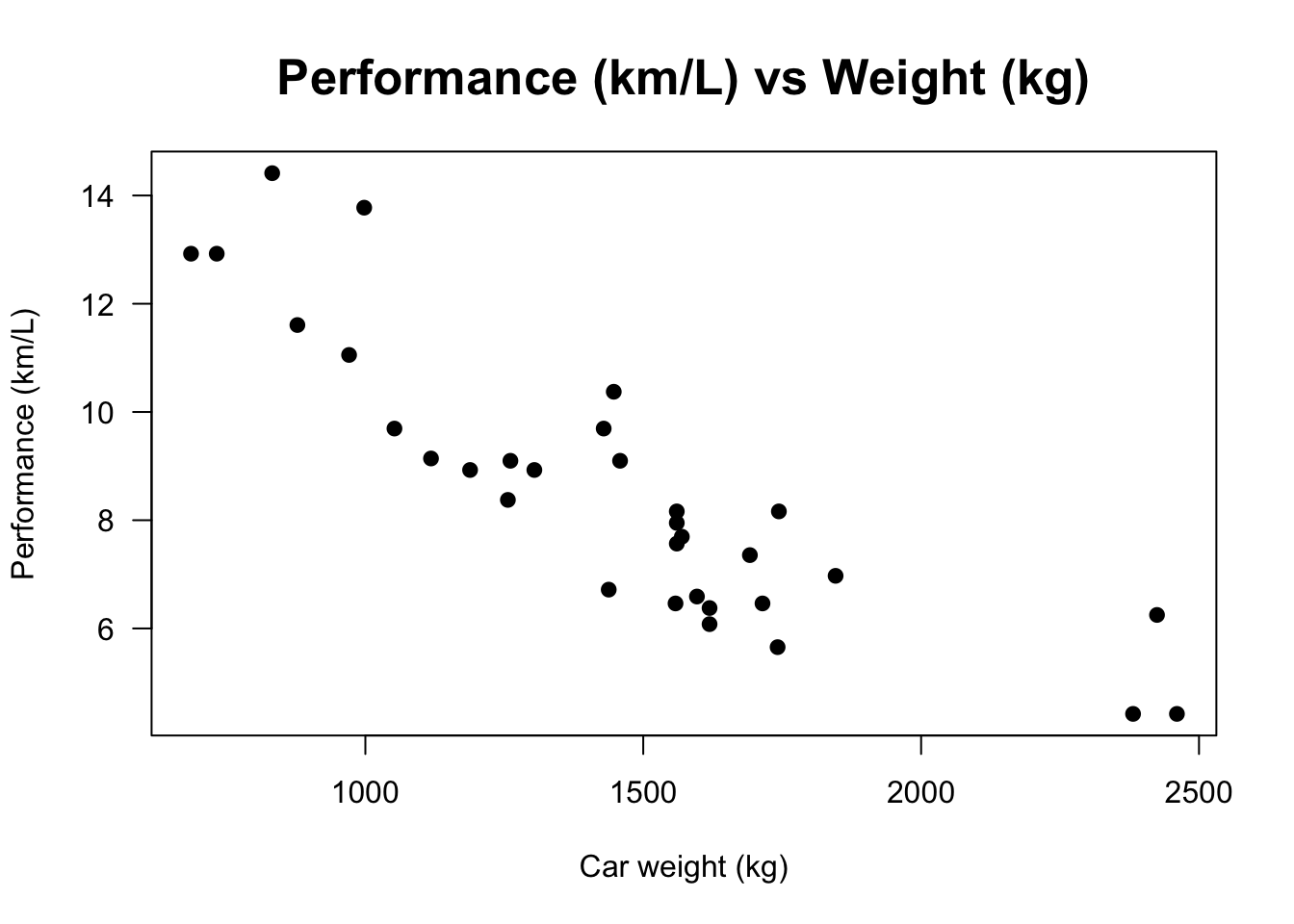# devtools::install_github("kassambara/ggcorrplot")
library(ggplot2)
library(ggcorrplot)

# Correlation matrix
data(mtcars)
corr <- round(cor(mtcars), 1)

# Plot
ggcorrplot(corr, hc.order = TRUE,
type = 'lower',
lab = TRUE,
lab_size = 3,
method = 'circle',
colors = c('tomato2', 'white', 'springgreen3'),
title = 'Correlogram of mtcars',
ggtheme = theme_bw)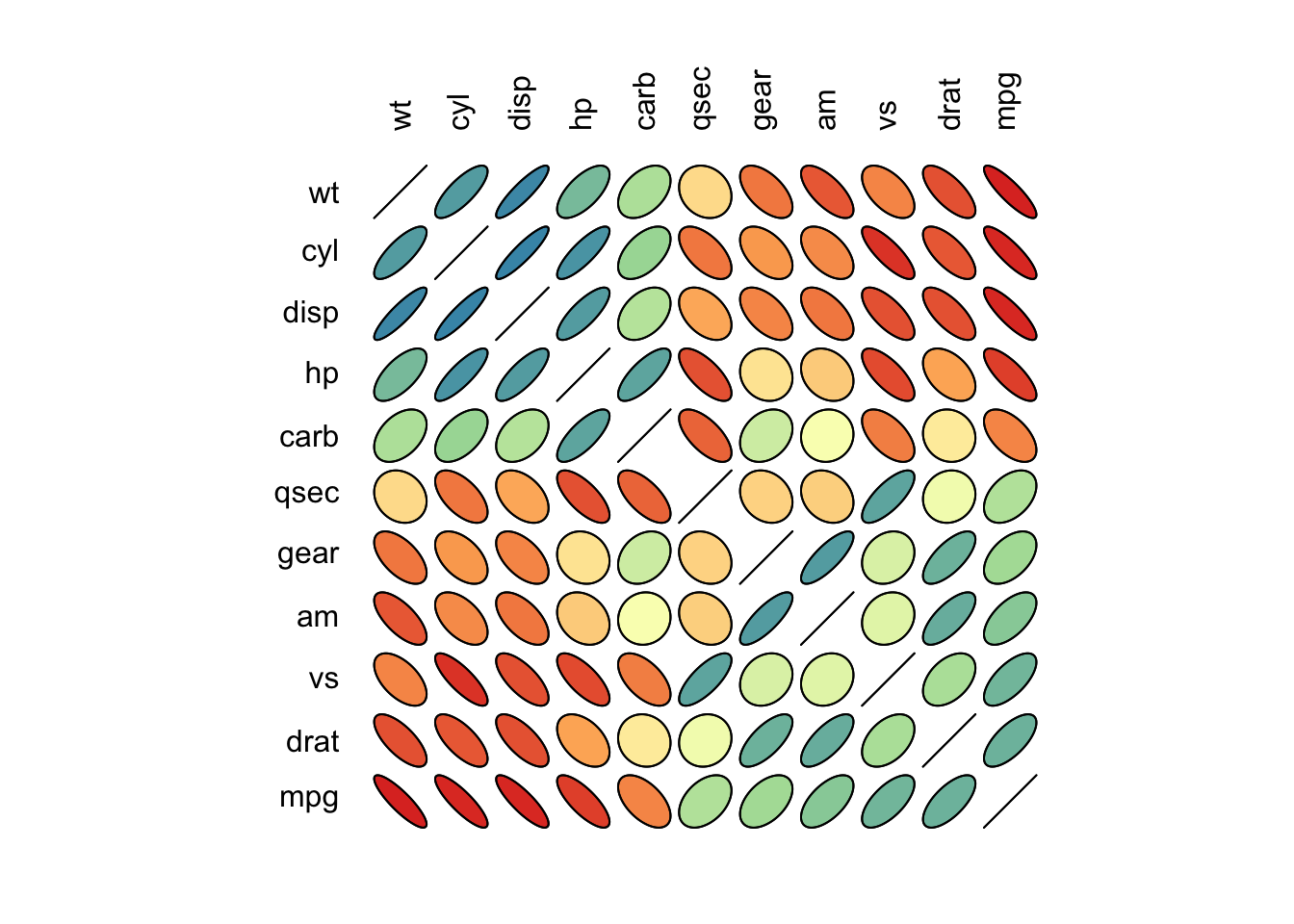However there is an important distinction between a cookbook and a restaurant menu. The latter presents choices among complete dishes in each of which the individual flavors are blended and disguised. The former — and this book — reveals the individual ingredients and explains how they are prepared and combined. (W. H. Press et al. 1992, xiv)

### References

Benjamini, Yoav. 1988. “Opening the Box of a Boxplot.” The American Statistician 42 (4): 257–62. https://www.tandfonline.com/doi/abs/10.1080/00031305.1988.10475580.
Cohen, I Bernard. 1984. “Florence Nightingale.” Scientific American 250 (3): 128–37. https://www.jstor.org/stable/pdf/24969329.pdf.
Cook, Edward. 1913. The Life of Florence Nightingale. The Macmillan Company. https://ia801504.us.archive.org/24/items/in.ernet.dli.2015.64009/2015.64009.The-Life-Of-Florence-Nightingale.pdf.
Esty, Warren W, and Jeff Banfield. 2003. “The Box-Percentile Plot.” Journal of Statistical Software 8: 1–14. https://doi.org/10.18637/jss.v008.i17.
Kopf, Edwin W. 1916. “Florence Nightingale as Statistician.” Quarterly Publications of the American Statistical Association 15 (116): 388–404. https://www.jstor.org/stable/pdf/2965763.pdf.
McGill, Robert, John W Tukey, and Wayne A Larsen. 1978. “Variations of Box Plots.” The American Statistician 32 (1): 12–16. https://www.tandfonline.com/doi/pdf/10.1080/00031305.1978.10479236.
Nightingale, Florence. 1858. “Notes on Matters Affecting the Health, Efficiency, and Hospital Administration of the British Army.” London: Printed by Harrison and Sons, St. Martin’s Lane, W.C. https://archive.org/details/b20387118.
Pearson, Karl. 1895. “X. Contributions to the Mathematical Theory of Evolution.—II. Skew Variation in Homogeneous Material.” Philosophical Transactions of the Royal Society of London.(A.), no. 186: 343–414. https://doi.org/10.1098/rsta.1895.0010.
Press, William H., Saul Teukolsky, William T. Vetterling, and Brian P. Flannery. 1992. Numerical Recipes in C: The Art of Scientific Computing. Cambridge University Press. https://www.grad.hr/nastava/gs/prg/NumericalRecipesinC.pdf.
Scott, David W. 1979. “On Optimal and Data-Based Histograms.” Biometrika 66 (3): 605–10. http://materias.df.uba.ar/mtb2019c1/files/2014/08/On-optimal-and-Data-based-histograms.pdf.
Tufte, Edward R. 1993. “Envisioning Information.”
———. 2006. Beautiful Evidence. Graphis Pr.
———. 2020. Seeing with Fresh Eyes: Meaning, Space, Data, Truth. Graphics Press LLC.
Tufte, Edward R, and Peter R Graves-Morris. 1983. The Visual Display of Quantitative Information. Vol. 2. 9. Graphics press Cheshire, CT. https://www.cs.rug.nl/svcg/uploads/VisualAnalytics/Tufte01-2.pdf.
Tufte, Edward R, Susan R McKay, Wolfgang Christian, and James R Matey. 1998. “Visual Explanations: Images and Quantities, Evidence and Narrative.” American Institute of Physics.
Tukey, John W. 1977. Exploratory Data Analysis. Addison-Wesley Publishing Company.
Walker, Francis Amasa. 1874. The Indian Question. Boston: JR Osgood. https://www.gutenberg.org/files/27058/27058-h/27058-h.htm.
Wilkinson, Leland. 2005. The Grammar of Graphics, 2nd Edition. Springer.
Zabala, Filipe J. 2009. “Desempate Técnico.” Master’s thesis, Universidade de São Paulo. https://doi.org/10.11606/D.45.2009.tde-01032021-140004.